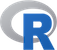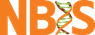@# Introduction

Even though plotting capabilities of R base are really impressive compared to other programming languages, there are other packages available to help you generate awesome graphics. Two of the more popular packages besides the base package are lattice and ggplot2. According to many users, these are superior to the base plot library, especially when it comes to exploratory data analysis – without too much work, they generate trellis graphics, e.g. graphs that display a variable or the relationship between variables, conditioned on one or more other variables. Over the last years ggplot2 has become the standard plotting library for many R users, especially as it keeps evolving and new features are added continuously. In addition to being more convient for certain types of plots, many feel that the default colors, axis types etc. look better on ggplot2 compared to the base R and lattice libraries.

# Exercise

This exercise will not consist of particular tasks for you to solve. Instead, the code blocks below will read in data and generate map plots. Make sure that you can run the code and generate the plot. Once you have a working code go over the code line by line and try to obtain a fairly detailed understanding of what is going on. Read the help for some of the functions and try to replot things with altered arguments (e.g. colors or point shapes or replot data for Lund instead of Uppsala).

Before you start you should download the following files to your working directory:
Bolaget.csv
coop_Uppsala.kml
ica_Uppsala.kml

If you cannot load the library install it either via the menu of RStudio or by typing `install.packages("ggmap")` in R.

## IMPORTANT!

In order to be able to install the rgdal library, you have to have the gdal software installed on your computer. OsX users who use Homebrew can do this by going to Terminal and typing brew install gdal. Other users who want to have this installed can follow this link: http://trac.osgeo.org/gdal/wiki/DownloadingGdalBinaries However, the rgdal library is used in this lab for just one purpose: to convert from RT90 to WGS84 datum in order to display coordinates prroperly on Google Map. The original Bolaget.csv file has coords in RT90 only, but we added 2 extra columns with the corresponding WGS84 coords. If you, however, feel like installing rgdal and doing conversion yourself, uncomment the #rgdal lines and comment the coords <- line.

``````# Load the libraries necessary for working with maps
# Install them if missing
library(ggmap)
library(maptools)
library(sp)
# library(rgdal) #rgdal
library(deldir)

# Download the map of Uppsala from Stamen Maps
city.coords <- c(17.63,59.84) # coordinates of the city in WGS84 datum. Here, Uppsala.
google.map <- get_map(city.coords, zoom=12, maptype = 'toner')

# Load the list of System Bolaget shops

# Narrow down the list to Uppsala only or any other city you want.
# If you change the city, remember to load new maps centered around your city
# with new city.coords
data <- data[data\$Address4 == "UPPSALA",]

# The description in the file says the provided coords are in the RT90 datum.
# The map uses WGS84 thus we need a conversion from RT90 to WGS84:
#latlonRT90 <- data[,c('RT90x', 'RT90y')] #rgdal
# colnames(latlonRT90) <- c('x','y') #rgdal

# EPSG codes for RT90 and WGS84 are 3021 and 4326, respectively.
# Here, we do the actual conversion
#tmp <- data.frame(coords.x = latlonRT90\$y, coords.y = latlonRT90\$x) #rgdal
#coordinates(tmp)=~coords.x+coords.y #rgdal
#proj4string(tmp)=CRS("+init=epsg:3021") #rgdal
#coords <- spTransform(tmp, CRS("+init=epsg:4326")) #rgdal

# Create the data frame for ggplot2
# coords <- data.frame(lat=coords@coords[,1], lon=coords@coords[,2]) #rgdal
coords <- data.frame(lat=data\$WGS84y, lon=data\$WGS84x) # turn off if using rgdal

# Do the Voronoi tesseleation
voronoi <- deldir(coords)

# Plot the map, shops density, shops as points and the tesselation lines.
map +
stat_density2d(data = coords,
aes(x = lat, y = lon,
fill = ..level..,
alpha = ..level..),
size = 0.01, bins = 50,
geom = "polygon") +
scale_fill_gradient(low = "green", high = "olivedrab", guide = FALSE) +
scale_alpha(range = c(0, 0.1), guide = FALSE) +
geom_segment(aes(x = x1, y = y1, xend = x2, yend = y2),
size = .6, linetype=2, data = voronoi\$dirsgs, color= "olivedrab") +
geom_point(aes(x=lat, y=lon), data=coords, color='olivedrab')

# ICA & Coop
ica.kml <- getKMLcoordinates(kmlfile="ica_Uppsala.kml", ignoreAltitude=T)
tmp <- unlist(ica.kml)
ica.coords <- data.frame(lat=tmp[1:length(tmp) %% 2 == 0], lon=tmp[1:length(tmp) %% 2 == 1], type='ica')
coop.kml <- getKMLcoordinates(kmlfile="coop_Uppsala.kml", ignoreAltitude=T)
tmp <- unlist(coop.kml)
coop.coords <- data.frame(lat=tmp[1:length(tmp) %% 2 == 0], lon=tmp[1:length(tmp) %% 2 == 1], type='coop')
coords <- rbind(ica.coords, coop.coords)
google.map <- get_map(c(17.63,59.84), zoom=12)
voronoi <- deldir(coords)